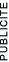# Règles IUPAC (Green Handbook) - Unités SI

Abbreviated list of quantities, units and symbols in physical chemistry

5. Units Outside the SI

6. Values of Some Fundamental Constants
7. References

1. Base SI units and physical quantities

A physical quantity is the product of a numerical value (a pure number) and a unit.

Physical quantities are organized in a dimensional system built upon seven base quantities. The International System of Units (SI) is based on the seven base units having the same dimensions as the associated physical quantities. Their names and symbols are as follows:

 Base Physical Quantity Symbol for Quantity Name of SI Unit Symbol for SI Unit length l metre m mass m kilogram kg time t second s electric current I ampere A thermodynamic temperature T kelvin K amount of substance n mole mol luminous intensity Iv candela cd

The symbol for a physical quantity is a single letter of the Latin or Greek alphabet printed in italic (sloping) type. It may be modified by subscripts and/or superscripts of specified meaning, or further characterized in particular cases through annotations in parenthesies put directly behind the symbol. The symbol for a unit is printed in roman (upright) type. Neither symbol should be followed by a full stop (period).

The physical quantity 'amount of substance' or 'chemical amount' is proportional to the number of elementary entities - specified by a chemical formula - of which the substance is composed. The proportionality factor is the reciprocal of the Avogadro constanct L (6.022 x 1023 mol-1). The amount of substance should no longer be called 'number of moles'.

Examples of relations between "amount of substance" and other physical quantities:

2 moles of N2 contain 12.044-x-1023 molecules of N2, amount of N2 = n(N2) = number of N2 molecules/L;

1.5 moles of Hg2Cl2 have a mass of 708.13 g;

1 mole of photons with frequency 1014 Hz has an energy of 39.90 kJ;

1 mole of electrons, e-, contains 6.022-x-1023 electrons, has a a mass of 5.468-x-10-7 kg, and a charge of -96.49 kC.

2. SI prefixes

Prefixes to form the names and symbols of the decimal multiples and submultiples of SI units.*

 Multiple Prefix Symbol 10-24 yocto y 10-21 zepto z 10-18 atto a 10-15 femto f 10-12 pico p 10-9 nano n 10-6 micro µ 10-3 milli m 10-2 centi c 10-1 deci d 10 deca da 102 hecto h 103 kilo k 106 mega M 109 giga G 1012 tera T 1015 peta P 1018 exa E 1021 zeta Z 1024 yotta Y

* Decimal multiple and submultiples of the unit of mass are formed by attaching prefixes to gram, examples: mg, not µkg; Mg, not kkg.

3. Examples of SI derived units with special names and symbols

 Physical Quantity (for symbols see Sect. 4 ) Name of SI Unit Symbol for SI Unit Expression in Terms of SI Base Units frequency hertz Hz s-1 force newton N m kg s-2 pressure, stress pascal Pa m-1 kg s-2 (= N-m-2 ) energy, work, heat joule J m2 kg s-2 (= N-m = Pa-m3 ) power watt W m2 kg s-3 (= J-s-1) electric charge coulomb C s A electric potential volt V m2 kg s-3 A-1 (= J-C-1) electric capacitance farad F m-2 kg-1 s4 A2 (= C-V-1) electric resistance ohm W m2 kg s-3 A-2 (= V-A-1) electric conductance siemens S m-2 kg-1 s3 A2 (= W-1) magnetic flux weber Wb m2 kg s-2 A-1 (= V s) magnetic flux density tesla T kg s-2 A-1 (= V s m-2) inductance henry H m2 kg s-2 A-2 (= V A-1 s) Celsius temperature* degree Celsius oC K plane angle radian rad 1 ** (rad and sr may be included or omitted in expressions for the derived units) solid angle steradian sr 1 **

* The Celsius temperature is defined by q / oC = T / K-273.15

4.1 physical quantities: space and time

 Physical Quantity Symbol SI Unit Cartesian space coordinates x, y, z m position vector r m length l m height h m thickness, distance d, d m radius r m diameter d m path, length of arc s m area A, As, S m2 volume V m3 plane angle a, b, g, q, f 1, rad solid angle w, W 1, sr time t s frequency v, f Hz angular frequency (=2pv) w s-1, rad s-1 characteristic time interval, relaxation time, time constant t, T s velocity v , u , w , c m s-1 acceleration a m s-2

4.2 physical quantities: mechanics

 Physical Quantity Symbol SI Unit mass m kg reduced mass µ kg (mass) density r kg m-3 relative density d 1 specific volume v m3 kg-1 moment of inertia I kg m2 momentum p kg m s-1 angular momentum L kg m2 s-1 rad (= J s) force F N (= kg m s-2) weight G, W N pressure p, P Pa surface tension g, s N m-1, J m-2 energy E J potential energy Ep , V, F J kinetic energy Ek , T, K J work w, W J Hamilton function H J power P W

4.3 physical quantities: general chemistry

 Physical Quantity Symbol SI Unit number of entities N 1 amount of substance, chemical amount n mol molar mass M kg mol-1 relative molecular mass, (molecular weight) Mr 1 relative atomic mass, (atomic weight) Ar 1 molar volume Vm m3 mol-1 mass fraction w 1 volume fraction f 1 mole fraction x, y 1 partial pressure of substance B p B Pa number concentration C, n m-3 amount (of substance) concentration of B or concentration of B c B , [ B ] mol m-3 molality m mol kg-1 mass concentration r, g kg m-3 surface concentration G mol m-2 stoichiometric number v 1 extent of reaction x mol

4.4 physical quantities: chemical kinetics

 Physical Quantity Symbol SI Unit rate of conversion x mol s-1 rate of concentration change of substance B (through chemical reaction) v B , r B mol m-3 s-1 rate of reaction v mol m-3 s-1 overall order of reaction n 1 rate constant, rate coefficient k (m3 mol-1)n-1 s-1 half life t1/2 s pre-exponential factor A (m3 mol-1)n-1 s-1 energy of activation E, Ea J mol-1 Gibbs energy of activation DG J mol-1 collision cross section s m2 collision frequency(of A with A ) zA (A) s-1 collision frequency factor zAB m3 mol-1 s-1 quantum yield, photochemical yield f, F 1

4.5 physical quantities: atoms, molecules and spectroscopy

 Physical Quantity Symbol SI Unit nucleon number, mass number A 1 proton number, atomic number Z 1 neutron number N 1 decay constant l s-1 ionization energy Ei , I J dissociation energy Ed , D J electron affinity Eea J quantum numbers: principal n 1 electron orbital l, L 1 -- component ml , ML 1 electron spin s, S 1 -- component ms , MS 1 total angular momentum j, J 1 -- component mj , MJ 1 nuclear spin I 1 -- component MI 1 vibrational v 1 magnetic moment of a particle µ, m J T-1, A m2 magnetogyric ratio g C kg-1 g-factor g 1 Larmor circular frequency wL s-1 quadrupole moment Q,, Q, eQ C m2 wavelength l m wavelength in a vacuum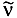m-1 total term T m-1 electronic term Te m-1 vibrational term G m-1 rotational term F m-1 rotational constants A, B, C m-1

4.6 physical quantities: electricity and magnetism

 Physical Quantity Symbol SI Unit electric charge Q C charge density r C m-3 electric current I A electric current density j A m-2 electric potential f, V V electric potential difference, voltage U, Df, DV V electric field strength E V m-1 electric displacement D C m-2 capacitance C F permittivity e F m-1 relative permittivity er 1 dielectric polarization P C m-2 electric susceptibility ce 1 polarizability (of a particle) a m2 C V-1 electric dipole moment p, µ C m magnetic flux F Wb Magnetic flux density B T magnetic field strength H A m-1 permeability µ H m-1, N A-2 relative permeability µr 1 magnetization M A m-1 magnetic susceptibility c 1 molar magnetic susceptibility cm m3 mol-1 resistance R W conductance G S resistivity r W m conductivity k S m-1 self-inductance L H

4.7 physical quantities: thermodynamics and statistics

 Physical Quantity Symbol SI Unit heat q, Q J work w, W J thermodynamic temperature T K Celsius Temperature q, t oC internal energy U J enthalpy H J standard reaction enthalpy D r H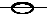J mol-1 entropy S J K-1 Gibbs energy G J Helmholtz energy A J heat capacity C J K-1 ratio Cp / Cv g, k 1 Joule-Thomson coefficient µ, µJT K Pa-1 isothermal compressibility k Pa-1 cubic expansion coefficient a K-1 chemical potential µ J mol-1 affinity of a reaction A J mol-1 fugacity f,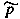Pa (relative) activity a 1 activity coeficient: -- mixtures f 1 -- solutes g 1 osmotic coefficient f 1 osmotic opressure P Pa equilibrium constant K, K 1 -- on a concentration basis Kc (mol m-3)Sv -- on a pressure basis Kp PaSv -- on a molality basis Km (mol kg-1)Sv density of (energy) states r(E) J-1 statistical weight g 1 partition function: -- particle q, z 1 -- canonical ensemble Q, Z 1 -- microcanonical ensemble W 1 symmetry number s, s 1 charateristic temperature Q K

 Physical Quantity Symbol SI Unit radiant energy Q, W J radiant intensity I, Ie W sr-1, W emissivity, emittance e 1 absorptance a 1 reflectance r 1 transmittance t 1 absorption coefficient: -- linear (decadic) a m-1 -- molar (decadic) e m2 mol-1 refractive index n 1 molar refraction Rm m3 mol-1 angle of optical rotation a 1, rad

4.9 Physical quantities: electrochemistry

 Physical Quantity Symbol SI Unit charge number of and ion z 1 ionic strength Ic mol m-3 Im mol kg-1 electromotive force, electrode potential E V electrochemical potential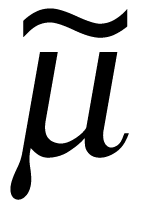J mol-1 overpotential h V pH pH 1 charge number of a cell reaction n, z 1 electrokinetic potential z V molar conductivity (of an electrolyte) L S m2 mol-1 molar conductivity (of an ion), ionic conductivity l S m2 mol-1 electric mobility u m2 V-1 s-1 transport number t 1

4.10 physical quantities: transport properties

 Physical Quantity Symbol SI Unit flux of a quantity X Jx , J (varies) mass flow rate qm,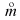kg s-1 volume flow rate qV,m3 s-1 heat flow rate F W thermal conductivity k, l W m-1 K-1 coefficient of heat transfer h W m-2 K-1 thermal diffusivity a m2 s-1 diffusion coefficient D m2 s-1 vicosity h, µ Pa s kinematic viscosity v m2 s-1

5.1 units outside the SI: units used with the SI

 Physical Quantity Unit Symbol for the Unit Value in SI Units SI Unit time minute min 60 s time hour h 3600 s time day d 86 400 s plane angle degree o (p/180) rad volume litre l, L 10-3 m3 mass tonne t 103 kg length angstrom Å 10-10 m pressure bar bar 105 Pa energy electronvolt* eV 1.60218 x 10-19 J mass unified atomic mass unit* u 1.660 54 x 10-27 kg

5.2 Other Units

These units were used in older literature. They are given here for the purpose of identification and conversion to SI units.

 Physical Quantity Unit Symbol for Unit Value in SI Units SI Units force dyne dyn 10-5 N pressure standard atmosphere atm 101325 Pa torr (mmHg) Torr 133.322 Pa energy erg erg 10-7 J thermochemcal calorie calth 4.184 J magnetic flux density gauss G 10-4 T electric dipole moment debye D 3.335 64 x 10-30 Cm viscosity poise P 10-1 N s m-2 kinematic viscosity stokes St 10-4 m2 s-1

6. Values of Some Fundamental Constants #

 Physical Quantity Symbol for Unit Value in SI Units Unit permeability of vacuum* µo 4p x 10-7 N A-2 speed of light in vacuum* co 299 792 458 m s-1 permittivity of vacuum eo 8.854 187 816 x 10-12 F m-1 elementary charge e 1.602 177 33(49) x 10-19 C Planck constant h 6.626 0755(40) x 10-34 J s Avogadro constant L, NA 6.022 1367(36) x 1023 mol -1 rest mass of electron me 9.109 3897(54) x 10-31 kg rest mass of proton mp 1.672 6231(10) x 10-27 kg Faraday constant F 9.648 5309(29) x 104 C mol-1 Hartree energy Eh 4.359 7482(26) x 10-18 J Bohr radius ao 5.291 772 49(24) x 10-11 m Bohr magneton µB 9.274 0154(31) x 10-24 J T-1 nuclear magneton µN 5.050 7866(17) x 10-27 J T-1 Rydberg constant R10 973 731.534(13) m-1 gas constant R 8.314 510(70) J K-1 mol-1 Boltzmann constant k, kB 1.380 658(12) x 10-23 J K-1 gravitational constant G 6.672 59(85) x 10-11 m3 kg-1 standard acceleration due to gravity* gn 9.806 65 m s-2 triple point of water* Ttp(H20) 273.16 K zero of Celsius scale* T(0oC) 273.15 K molar volume of ideal gas (at 1 bar and 273.15 K) Vo 22.711 08(19) L mol-1

#Cohen, E.R. and Taylor, B.N., J. Res. Nat. Bur. Stand. 92 (1987) 85-95.
*These values are exact by definition

7. References

Mills., I., Cvitas, T., Homann, K., Kallay, N. and Kuchitsu, K., Quantities, Units and Symbols in Physical Chemistry , 2nd edn. Blackwell Scientific Publications, Oxford 1993. (ISBN 0-632-03583-8.)

ISO Standards Handbook 2, Quantites and Units, ISO, Geneva 1993.

Cohen, E.r. and Giacomo, P., Symbols, Units Nomenclature and Fundamental Constants in Physics, 1987 Revision, Physica 146A (1987) 1-68.

Bureau International des Poids et Mesures, Le Systéme International d'Unités (SI), 6th French ad English Edition, BIPM, Sévres 1991.

 Copyright (c) 2000 - 2023 |  Miseur Ludovic | www.lachimie.net | tous droits réservés |  remis à jour le : 06/07/2023 Comment citer cette page : Miseur, L. (2023). Lachimie.net - cours de chimie et ressources didactiques pour apprendre les savoirs disciplinaires, les savoir-faire et compétences de base des sciences chimiques. La Chimie.net. http://www.lachimie.net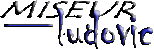Copyright © Miseur Ludovic  2000 - 2023 | www.educations.net | tous droits réservés |  remis à  jour le : 06/07/2023 La reproduction du site, même partielle, est formellement interdite sans accord écrit préalable de l'auteur.  plus d'infos : www.assucopie.be Comment citer ce site: Miseur, L. (2023). La Chimie.net. Consulté le DD/MM/YYYY sur http://www.lachimie.net